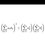# Interesting construction problems

Here are some interesting construction problems that I stumbled upon.

In the following problems, constructions can only be done using an unmarked straightedge and compass.

I came across the first problem accidentally while watching my friend fold origami. However, it might have been posed long ago by some mathematician. The third problem is easier than the others.

1. Given a line segment of length $l$ and any positive integer $n$. Show that, using straightedge and compass, it is always possible to divide the line into $n$ equal segments, no matter what $n$ is.

2. Given a line segment of length $\sqrt{2}+\sqrt{3}+\sqrt{5}$, is it possible to construct a line segment of length $1$?

3. Given triangle ABC, construct the circumcircle, and the incircle.

4. Given the perpendicular from A and two medians from A, B onto BC, AC respectively, reconstruct triangle ABC.Note by Joel Tan
6 years, 9 months ago

This discussion board is a place to discuss our Daily Challenges and the math and science related to those challenges. Explanations are more than just a solution — they should explain the steps and thinking strategies that you used to obtain the solution. Comments should further the discussion of math and science.

When posting on Brilliant:

• Use the emojis to react to an explanation, whether you're congratulating a job well done , or just really confused .
• Ask specific questions about the challenge or the steps in somebody's explanation. Well-posed questions can add a lot to the discussion, but posting "I don't understand!" doesn't help anyone.
• Try to contribute something new to the discussion, whether it is an extension, generalization or other idea related to the challenge.
• Stay on topic — we're all here to learn more about math and science, not to hear about your favorite get-rich-quick scheme or current world events.

MarkdownAppears as
*italics* or _italics_ italics
**bold** or __bold__ bold
- bulleted- list
• bulleted
• list
1. numbered2. list
1. numbered
2. list
Note: you must add a full line of space before and after lists for them to show up correctly
paragraph 1paragraph 2

paragraph 1

paragraph 2

[example link](https://brilliant.org)example link
> This is a quote
This is a quote
    # I indented these lines
# 4 spaces, and now they show
# up as a code block.

print "hello world"
# I indented these lines
# 4 spaces, and now they show
# up as a code block.

print "hello world"
MathAppears as
Remember to wrap math in $$ ... $$ or $ ... $ to ensure proper formatting.
2 \times 3 $2 \times 3$
2^{34} $2^{34}$
a_{i-1} $a_{i-1}$
\frac{2}{3} $\frac{2}{3}$
\sqrt{2} $\sqrt{2}$
\sum_{i=1}^3 $\sum_{i=1}^3$
\sin \theta $\sin \theta$
\boxed{123} $\boxed{123}$

## Comments

Sort by:

Top Newest

2: Follows directly from the definition of a constructible number.

Constructively, show that $\sqrt{n}$ is always constructible when $n \in \mathbb{Z}^{+}$ and use compass equivalence theorem to add them up.

3: Let $O$ be the circumcenter of $\Delta ABC$. It's pretty obvious that $\Delta AOB$ is isoceles, and that the angle bisector of $\angle AOB$ is the perpendicular bisector of $AB$; hence, by similar argument for the other sides, $O$ is the intersection of the perpendicular bisectors of the sides of $\Delta ABC$. Circumcircle construction is just a corollary.

Let $O^{\prime}$ be the incenter of $\Delta ABC$. Let the shortest distance from the incenter to $AB$ intersect the latter at $X$; do similarly for $AC$, with the intersection $Y$. $O^{\prime}X \equiv O^{\prime}Y$ (radii), and $AX \equiv AY$ (convergent tangents). It's easy to see the angle bisector of $\angle ABC$ passes through the incenter; thus, by similar argument for the other angles, $O^{\prime}$ is the intersection of the angle bisectors of the interior angles of $\Delta ABC$. Again, incircle is just a corollary (albeit a slightly more complicated one).

- 6 years, 5 months ago

Log in to reply

I'm new to compass and straightedge, so I'm sorry if I used any theorems incorrectly.

As for the incircle corollary, my method is to pick any side of $\Delta ABC$ and construct any circle with center $O^{\prime}$ that intersects that side. The median of the two intersections on the side will be a point on the circumference of the incircle.

- 6 years, 5 months ago

Log in to reply

×

Problem Loading...

Note Loading...

Set Loading...